Published: 30 December 2014

# Dynamic characteristics of vehicle-wheel/rail nonlinear contact-foundation system

Yaping Wu1
Yunpeng Wei2
Guiman Xu3
Zhidong Duan4
Liangbi Wang5
1, 2, 3, 4, 5Lanzhou Jiaotong University, Lanzhou 730070, China
Corresponding Author:
Yaping Wu
Views 166

#### Abstract

To study the dynamic characteristics of the vehicle-wheel/rail-foundation system of high-speed trains with consideration of nonlinear wheel/rail contact relationship and effects of nonlinear wheel/rail contact behavior on the railway rail, foundation structure and vehicle, a three-dimensional finite element model of vehicle-wheel/rail nonlinear contact-foundation system is established by using ANSYS software in which, the special contact element is used to simulate wheel/rail nonlinear contact and instantaneous separation. The vertical vibration of the whole system and its spread parts due to the track irregularities are calculated. At the same time, the relationships between the vibration of the wheel/rail system and the train speed, foundation stiffness are analyzed. The numerical results show that the appropriate foundation stiffness is conducive to weaken the vertical acceleration of the vehicle and wheel/rail system, and the vertical displacement and wheel/rail contact stress on the rail top increase with the growth of the train speed. The procedure and results from this paper can provide reference for the design of vehicle-wheel/rail system.

## 1. Introduction

Wheel/rail system is an important part of the railway transportation and its working environment is very complex. While the train is in motion, the vibration of the wheel/rail system will influence the foundation structure inevitably, in return, the deformation and vibration of foundation structure will also influence the vibration of the system. In recent years, many scholars have conducted a range of studies on this problem and published a series of research papers. Hamed Ronasi and Jens C. O. Nielsen  discussed and compared the three numerical algorithms for the identification of contact forces between wheel and rail caused by two load cases comprising rail irregularity induced excitation and transient excitation. João Pombo·Jorge Ambrosio  designed a method to get the realistic representation of a three-dimensional track, and its results show track irregularities will increase the contact force and cause the fracture damage to the track foundation and deteriorate the wheel and rail surface. Ahmed A. Shabana, et al.  presented the four nonlinear dynamic formulations to describe the contact behaviors of the wheel/rail, and the properties of the formulations were compared and their performance were evaluated. Hyun Jik Cho, et al.  established a nonlinear finite element model in which wheel-rail interfaces was simulated by the surface-to-surface elements to study the derailment mechanisms. R. U. A. Uzzal, et al.  simplified firstly a 17-degrees of freedom lumped mass vehicle as a nonlinear model, and than the contact loads on the wheel/rail contact point and corresponding dynamic response were presented, and results show that the train speed has an obvious effect on the wheel-rail impact force. Muzaffer Metin and Rahmi Guclu  presented an active vibration control method with comparative algorithms of half rail vehicle model under various track irregularities, in which the vertical motions of the vehicle and passenger seat were researched by taking into account of the two different track irregularities. M. Pau  proposed a new method, which employs frequently used device such as a standard NDE control, to investigate the contact characteristics between wheel and rail. S. C. Yang and E. Kim  used an effective experimental apparatus to test the effect of installation faults on vehicle and track interaction in the concrete bearing surface of a direct-fixation track. Pablo Antolín, et al.  studied the dynamic vehicle-bridge interaction in high-speed railways with consideration of nonlinear wheel-rail contact forces, in the procedure wheel was considered to be rigidly attached to the rail and a wheel-rail contact force was proposed.

Wheel/rail contact behavior is a highly nonlinear problem, and the geometries of wheel and rail are complex. The wheel/rail vibration is an especially complicated mechanical behaviour and the vibration system includes the wheel/rail, foundation, concrete sleeper, pad and vehicle. In the previous research, the vehicle-wheel/rail vibration system is usually broke down into two parts, one is the vehicle and the other is track and foundation, or in the system a Hertzian spring is used to connect wheel and rail, in addition track-sleeper-foundation structure could also be reduced, or even be simplified, in many cases, as a rigid body. Above methods can simplify the calculations, but difficult to simulate nonlinear dynamic contact behaviors between wheel and rail so the corresponding calculation results are not too accurate. Based on the above situation for the wheel/rail nonlinear contact-foundation system, more accurate analysis model and calculation method are still in development.

In view of the above questions, with consideration of elastic and elastoplastic properties of the material, a three-dimensional finite element model of vehicle-wheel/rail nonlinear contact-foundation system is established by using ANSYS software in which, the special contact elements are used to simulate wheel/rail nonlinear contact and instantaneous separation. Under the conditions in which the influences of the track structure vibration and deformation on wheel/rail rolling contact geometry relationship, contact force, and wheel/rail instantaneous separation can be considered, the responses of each part of the vehicle-wheel/rail nonlinear contact-foundation system, in different speeds and foundation stiffness, to the track irregularities are calculated and analyzed. The method and the results from this paper have reference significance to optimize design of wheel/rail system.

## 2. Finite element model and parameters

### 2.1. Finite element model of the system

The vehicle-wheel/rail nonlinear contact-foundation system is composed of sprung mass, vehicle suspension spring, axle, wheel, rail, rubber pad, concrete sleepers and foundation, as shown in Fig. 1.

Fig. 1Sketch map of wheel/rail system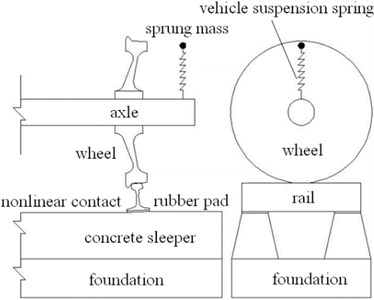Fig. 2Finite element model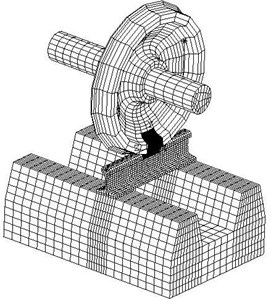This paper only considers the vertical vibration of the whole system caused by the track irregularities, so only the vertical vibration is calculated. In order to simplify the calculation, the vehicle is simplified as a mass point, the vehicle suspension spring is considered as linear springs, and the damping effect is ignored.

In order to research the 3D wheel/rail nonlinear contact vibration mechanisms and its impact on the foundation structure, ANSYS software  is used.

The 3D finite element model which includes axle, wheel, rail, rubber pad, concrete sleepers and foundation is shown in Fig. 2 (sprung mass and vehicle suspension spring are taken into accounted in the calculations). The wheel type is TB conical tread and its diameter is 915 mm, the rail type is CHN60. The rail bottom slope is 1:20. The distance between adjacent sleepers is 60 cm.

The main difficulty in the analysis of wheel/rail contact problem is that the contact behavior is changing with the load, materials, boundary conditions and other factors. Furthermore, the two contact surfaces may be in contact or separated and the contact process is unpredictable. ANSYS software provides a set of contact elements which is a solution to this problem. The wheel/rail contact force can be expressed as follows:

1
$P=\left\{\begin{array}{ll}0,& {u}_{n}>0,\mathrm{c}\mathrm{o}\mathrm{n}\mathrm{t}\mathrm{a}\mathrm{c}\mathrm{t}\mathrm{i}\mathrm{n}\mathrm{g},\\ {K}_{n}\bullet {u}_{n},& {u}_{n}<0,\mathrm{s}\mathrm{e}\mathrm{p}\mathrm{a}\mathrm{r}\mathrm{a}\mathrm{t}\mathrm{i}\mathrm{n}\mathrm{g},\end{array}\right\$

where ${K}_{n}$ is the normal contact stiffness; ${u}_{n}$ is the gap size of the wheel and rail contact surface.

In the procedure, CONTACT174 is selected as the contact elements and TARGE170 is taken as the target elements. To solve the problem of coordination of the two contact surfaces, the expansion Lagrangian method is chosen. This method will find the exact Lagrange multipliers, so that the contact stiffness of penalty function can be corrected in a series of iterative process. The penalty function of contact stiffness is determined by the wheel and rail materials. Using the above method the improper contact stiffness can be avoided.

In order to ensure the convergence and obtain the reliable calculation results, the element sizes of the contact area should be divided small enough. Due to the complex geometries of the train wheel, a large amount of elements and nodes will be produced if using the same fine mesh in all bodies. Thus the calculation time will be very long, even difficult to continue. To solve this problem, ANSYS software provides a multiple point constraint (MPC) method. This method can realize the connection among discontinuous mesh, freedom incompatible mesh and different type elements. In this paper, in order to reduce the number of nodes and ensure the accuracy of contact area, the SOLID186 element with 20 nodes is used in the contact region and the SOLID185 element with 8 nodes is selected in the other parts. In the connection parts, the MPC method is adopted (Fig. 3).

Fig. 3MPC contacts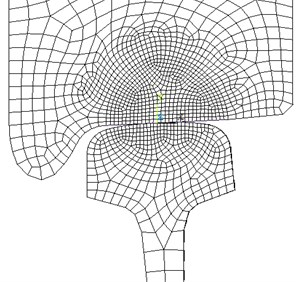Fig. 4The constitutive relationship of wheel/rail material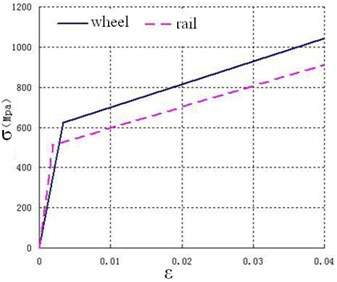### 2.2. Parameters

According to the reference , a single spring stiffness value is taken as 444 kN/m and two parallel springs connected with the vehicle are adopted, so the vehicle suspension spring stiffness is taken as 888 kN/m. Every rubber pad stiffness value is 100 MN/m. The elastic modulus of concrete sleepers is 39.8 GPa, and that of the axle is 207 GPa, the mass of vehicle is 8 t. The wheel and rail are elastic-plastic material. The bilinear kinematic hardening model is set as the constitutive model of wheel/rail material and their stress-strain relationship is shown in Fig. 4.

## 3. Excitation force due to track irregularity

The track irregularities are caused by many factors, such as the construction errors, the uneven settlement of the foundation and other factors. The rail surface vertical irregularities can be thought as a combination of several sine curves. In general, the rail irregularities can be expressed with three sine curves that correspond to long wave, medium wave and short wave irregularities, respectively. One section of track irregularity curve is shown in Fig. 5. The movement of the wheel can be seen as a simple harmonic motion with amplitude of ${a}_{i}$ and velocity of $v$ on the curve. At this moment, additional dynamic inertia force ${P}_{i}$ is applied to the wheel which can be given by:

2
${P}_{i}=M{a}_{i}{\omega }_{i}^{2},$

where $M$ denotes wheel quality ($M=$ 750 kg), ${a}_{i}$ denotes amplitude of the movement corresponding to the $i$th wave. ${\omega }_{i}$ denotes circular frequency corresponding to the $i$th wave and its formula is governed by:

3
${\omega }_{i}=\frac{2\pi v}{{L}_{i}},$

where $v$ denotes train speed and ${L}_{i}$ is the wavelength ($i=1,2,3$). The wavelength and its amplitude value are taken as follows: ${L}_{1}=$ 10 m, ${a}_{1}=$ 3.5 mm; ${L}_{2}=$ 2 m, ${a}_{2}=$ 0.4 mm; ${L}_{3}=$ 0.5 m, ${a}_{3}=$ 0.08 mm. Then the excitation force function can be given as follows :

4
$F\left(t\right)={P}_{0}+{P}_{1}\mathrm{sin}\left({\omega }_{1}t\right)+{P}_{2}\mathrm{sin}\left({\omega }_{2}t\right)+{P}_{3}\mathrm{sin}\left({\omega }_{3}t\right),$

where ${P}_{0}$ denotes wheel weight (${P}_{0}=$ 7.5 kN). The excitation force model of the rail irregularities is shown in Fig. 6.

Fig. 5Track irregularity curve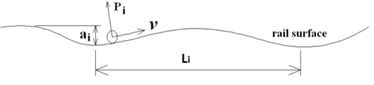Fig. 6Excitation force model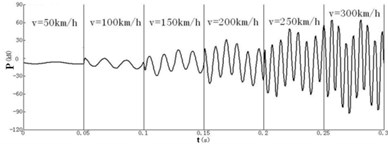## 4. Dynamic equation and solving

According to the dynamics theory, the vibration differential equation is governed by:

5
$\left[\mathbf{M}\right]\left[\stackrel{¨}{\mathbf{U}}\right]+\left[\mathbf{C}\right]\left[\stackrel{˙}{\mathbf{U}}\right]+\left[\mathbf{K}\right]\left[\mathbf{U}\right]=\left[\mathbf{F}\left(\mathbf{t}\right)\right],$

where $\left[\mathbf{M}\right]$, $\left[\mathbf{C}\right]$, $\left[\mathbf{K}\right]$, $\left[\mathbf{U}\right]$ and $\left[\mathbf{F}\left(\mathbf{t}\right)\right]$ are respectively mass matrix, damping matrix, stiffness matrix, displacement matrix and the external force vector.

Transient analysis includes the direct integration method and the mode superposition analysis. As the wheel/rail system is a highly nonlinear problem which includes material nonlinearity, geometric nonlinearity and contact state nonlinearity. Performing transient dynamic analysis by using ANSYS Software, the the dynamic response of the structural can be obtained. New-mark method is used for time integration in the solving procedure. The basic idea is, a set of discrete time points $0\le {t}_{i},{t}_{2},\dots ,{t}_{n}\le T$ is used to instead of a continuous time interval $\left[0,T\right]$. At each time interval $\left[{t}_{i},{t}_{i+1}\right]$, the displacement, velocity and acceleration are linear and the dynamic equation Eq. (5) should be satisfied at each time point. According to the initial conditions, backward integration step by step is carried to calculate the displacement response at each time point, then the velocity, acceleration, stress and strain can be obtained.

## 5. Numerical results

The rail irregularities excitation force $\left[\mathbf{F}\left(\mathbf{t}\right)\right]$ is applied to the system, thus the numerical results of dynamical response of the system can be provided. The following will be given some finite element calculation results fragment.

Fig. 7 shows the acceleration time history curves of the vehicle and rail top within 0.3 s time interval, while the elasticity modulus of foundation $E=$ 150 MPa and the train speed $v=$ 250 km/h. When time = 0.25 s the contour maps of displacement and acceleration are shown in Fig. 8.

Fig. 7Acceleration time history curves (E= 150 MPa, v= 250 km/h)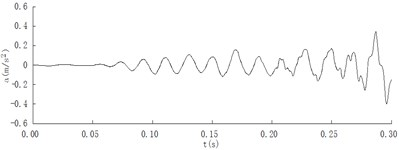a) Vehicle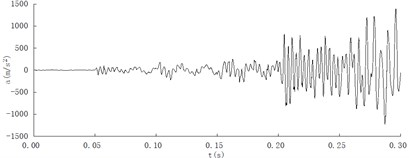b) Rail top

Fig. 8Contour maps of displacement and acceleration (t= 0.25 s, E= 150 MPa, v= 250 km/h)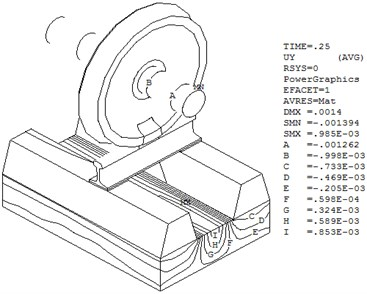a) Displacement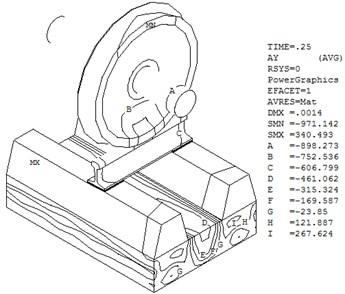b) Acceleration

Fig. 9Time history curve of contact stress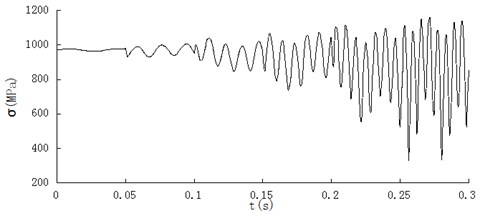Fig. 10Contour map of contact stress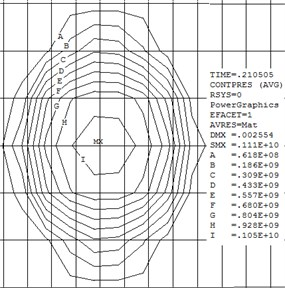Fig. 9 shows the time history curve of contact stress of wheel/rail while the elasticity modulus of foundation $E=$ 150 MPa and the train speed $v=$ 250 km/h. When time $=$ 0.21 s the contour map of contact stress on the wheel-rail contact point is shown in Fig. 10.

According to the numerical results of the dynamical response, we can analyze the dynamic characteristics of the system.

### 5.1. Displacement analysis

In the system, the vertical displacement of the vehicle ${U}_{C}$ can be expressed as follow:

6
${U}_{C}={U}_{h}+\sum _{i=1}^{7}{U}_{i},$

where ${U}_{h}$ is the compressive deformation of the vehicle suspension spring, ${U}_{1}$ is the bending deformation of the axle, ${U}_{2}$ is the elastic-plastic deformation of the wheel, ${U}_{3}$ is a local elastic-plastic deformation of the rail, ${U}_{4}$ is the bending deformation of the rail, ${U}_{5}$ is the amount of compressive deformation of the rubber pad, ${U}_{6}$ is the vertical compressive deformation of the concrete sleeper, ${U}_{7}$ is the foundation deformation.

### 5.1.1. The time history curves of the displacement components

Fig. 11 shows the time history curves of the displacement components of the wheel/rail system at different train speed. It can be seen that the values of compressive deformation of the vehicle suspension spring is maximum, followed by deformations of foundation and rubber pad. When the train speed is less than 200 km/h, the vertical displacement of rubber pad is greater than the compressive deformation of the foundation. But as the speed is greater than 200 km/h, this situation is just the opposite. Because the vehicle suspension spring can weaken the vibration, the displacement direction of the vehicle suspension spring is opposite to that of the other parts. Due to the elastic modulus of concrete sleeper is far greater than other parts its deformation is the smallest and can be ignored.

### 5.1.2. The effects of elastic modulus of foundation and train speed on the displacement

The peak displacement components are shown in Figs. 12-15. In above figures the effects of elastic modulus of foundation and train speed on the displacement value are displayed and it can be seen that displacement values increase with the increase of the train speed.

When train speeds achieve and exceed 200 km/h and the elastic modulus of the foundation exceed 15 MPa, the displacements on the wheel-rail contact point, suspension spring and foundation increases rapidly with the growth of the elastic modulus of foundation (see Figs. 12, 14, 15). And because the stiffness of the suspension spring is far less than the stiffness of other parts, which made the suspension spring to absorb most of the displacement, and so the displacements of the vehicle change relatively small (see Fig. 13).

When the elastic modulus of the foundation is 15 MPa. Because the foundation stiffness is too small the foundation displacement is too large, which results in the displacement of other parts being larger.

According to evaluates of dynamic characteristics of vehicle track interaction from the reference , the rail vertical displacement limit to keep the train stability is 2 mm. The above criterion requires that when the speed is lower than 300 km/h, the elastic modulus of foundation should be greater than 150 MPa (see Fig. 12).

Fig. 11Time history curves of the vertical displacement of the system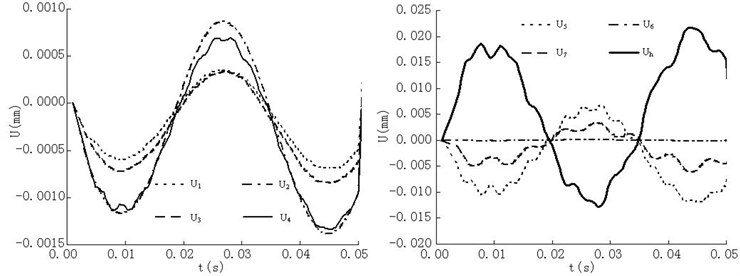a) Speed of $v=$ 50 km/h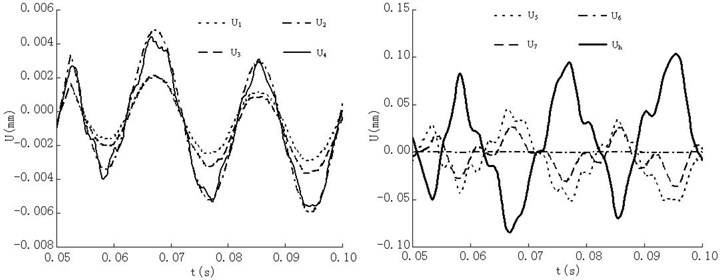b) Speed of $v=$ 100 km/h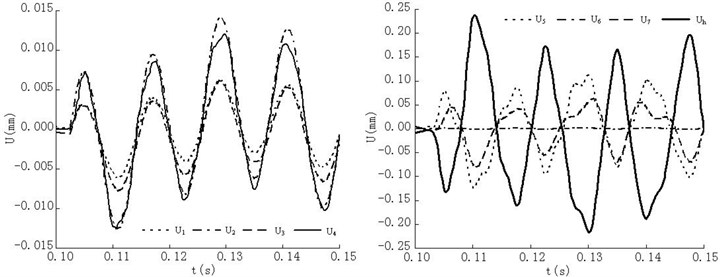c) Speed of $v=$ 150 km/h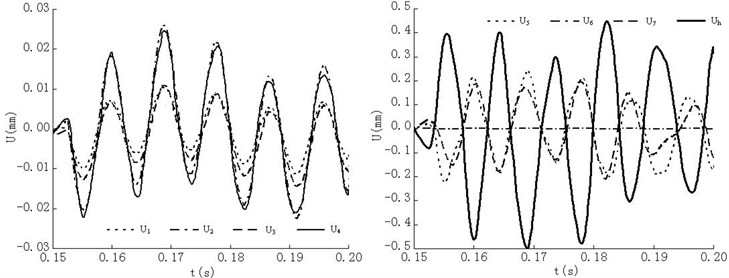d) Speed of $v=$ 200 km/h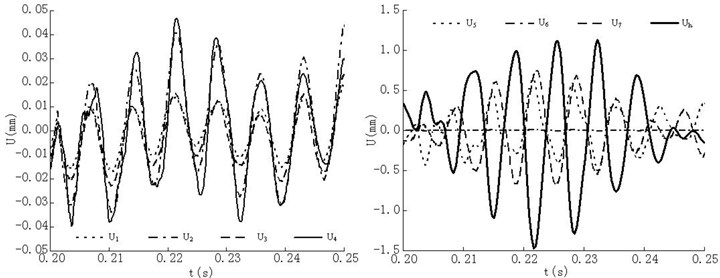e) Speed of $v=$ 250 km/h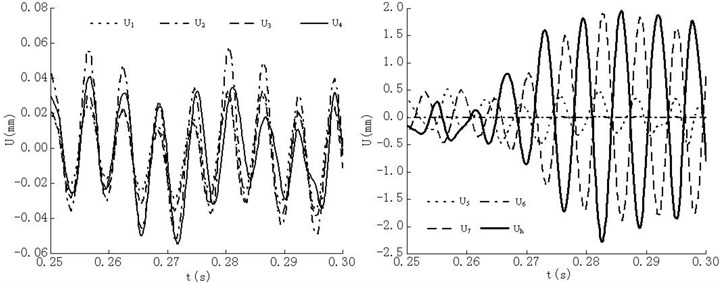f) Speed of $v=$ 300 km/h

Fig. 12Displacement of wheel-rail contact point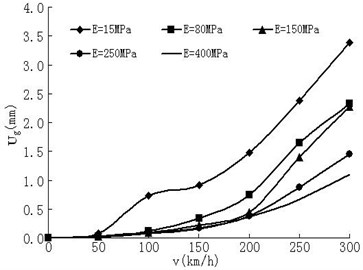Fig. 13Displacement of the vehicle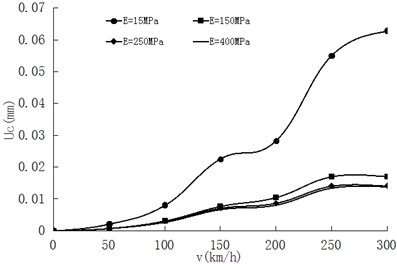Fig. 14Compression deformation of suspension spring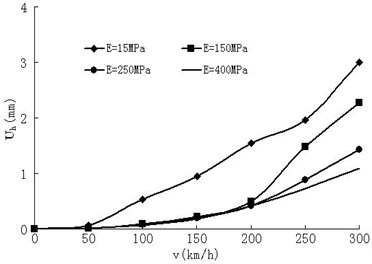Fig. 15Displacement value of the foundation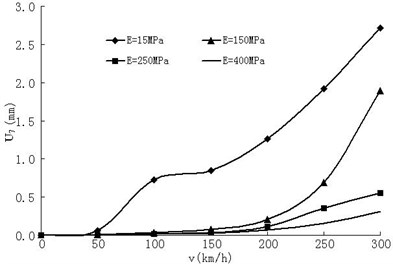From the above analysis, it can be concluded that selecting the appropriate elastic modulus of foundation ($E\ge$ 250 MPa) can effectively reduce the deformation of foundation, which also can improve the smoothness of track and the comfort of high speed train.

### 5.2. Acceleration analysis

Fig. 16 shows the relationships between the peak acceleration of center point located on the wheel/rail contact region and the train speed in different elastic modulus of the foundation. It can be seen that the differences of the acceleration values are very small in different elastic modulus when the speed is lower 150 km/h. But as the speed exceeds 150 km/h, the acceleration will increase with the growth of the elastic modulus of the foundation. At the speed of 300 km/h, vertical acceleration on the track contact spot increases firstly and then decreases while the elastic modulus increasing, and reach the maximum when elastic modulus is 150 MPa. According to evaluates of dynamic characteristics of vehicle track interaction from the reference , the rail vertical acceleration limit is 200 g. So the requirements can be met when the speed is lower than 250 km/h, or the elastic modulus of foundation is greater than 400 MPa.

Fig. 17 shows the vertical body acceleration variation. It can be seen that the vehicle body accelerations increase with the growth of train speed and the larger elastic modulus of the foundation in favor of reducing the vertical vibration acceleration and improving comfort of the vehicle.

Fig. 16Rail top acceleration variation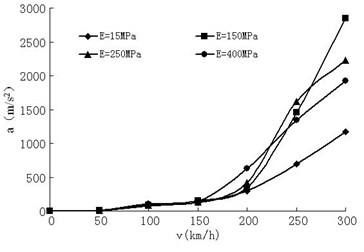Fig. 17Vehicle body acceleration variation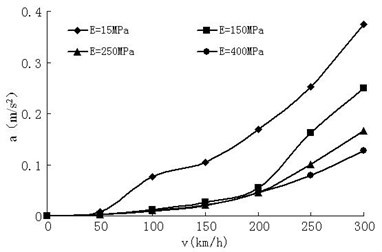### 5.3. Contact stress analysis

Fig. 18 shows the maximum contact stress of wheel/rail changes with the train speed. It can be seen that the contact stress increases with the speed increasing, and the variations of foundation elastic modulus have a weak effect on the contact stress.

Fig. 18The maximum contact stress vs speed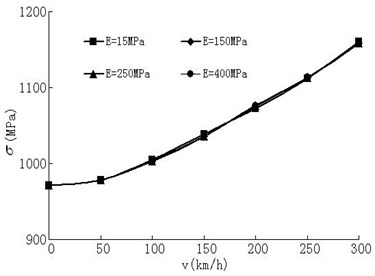Fig. 19The maximum contact stress vs elastic modulus at v= 250 km/h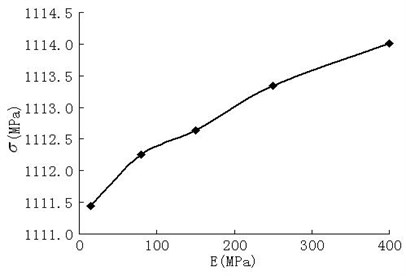Fig. 19. shows the relationship between the maximum contact stress and the elastic modulus of foundation at $v=$ 250 km/h. It can be seen that the contact stress increases with the growth of the foundation elastic modulus.

## 6. Conclusions

From above analysis, some conclusions can be drawn as following:

(1) The vehicle suspension spring deformation increases with the speed increasing and the elastic modulus (stiffness) decreasing. It played a key role in damping the vertical vibration of vehicle body.

(2) Displacement of track system increases with the speed increasing and substrate stiffness decreasing.

(3) The main part of the vehicle system displacement is suspension spring displacement, and the main part of the track foundation displacement is foundation displacement, the displacement direction of both is opposite, their synthesis results mainly determine the displacement of vehicle body.

(4) The smaller elastic modulus of foundation (foundation stiffness) decreases the top rail vertical vibration acceleration, but also increases the vertical acceleration, and reduces the vehicle comfort index. So, for the high-speed railway of vehicle speed over 200 km/h, higher foundation stiffness should be used as far as possible.

(5) The contact stress peak value between wheel and rail increases with the train speed rising. The different foundation elastic modulus has litter effect on the contact stress.

(6) The model and procedure from this paper can consider the influences of actual track structure vibration and elastic deformation on wheel/rail rolling contact geometry relationship, contact force, and the suggestion has reference significance to optimize the design of wheel rail system.